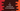# How to find the income tax of an employee in C## How to find the income tax of an employee in C:

In this C programming example, we will learn how to find the income tax of an employee in C. We will take some predefined tax-rules and find the income tax based on the basic salary of an employee.

With this program, you will learn how to do basic mathematical calculations in C, how to take user inputs in C and how to print data on console.

### Tax condition:

We will use the following rules to calculate the final income tax:

``````< 250000 : Nil
250000 to 500000 : 2.5%
500000 to 1000000 : 5%
above 1000000 : 15%``````

This is not a valid tax rules for any country. We are using this only for this example purpose. You can modify the program to any other income range and tax values for different ranges.

## C program to calculate income tax:

Below is the complete program that calculates the income tax of a given salary:

``````#include <stdio.h>

int main()
{
float salary, tax = 0;

printf("Enter the basic salary: ");
scanf("%f", &salary);

if (salary >= 1000000)
{
tax = salary * 15/100;
}
else if (salary >= 500000)
{
tax = salary * 5/100;
}
else if (salary >= 250000)
{
tax = salary * 2.5/100;
}

printf("Tax calculated: %0.2f\n", tax);

return 0;
}``````

Here,

• We have defined two float variables salary and tax to hold the salary and tax.
• It asks the user to enter the basic salary. It reads the salary and stores it in the salary variable.
• The if-else if block is checking the user entered value and based on it, it finds the tax.

If you run this program, it will print output as like below:

``````Enter the basic salary: 23499
Tax calculated: 0.00

Enter the basic salary: 34592
Tax calculated: 0.00

Enter the basic salary: 3458897
Tax calculated: 518834.56``````

## C program to calculate income tax using a separate function and Macros:

Let’s use a separate function that calculates the income tax and Macros to make it readable.

``````#include <stdio.h>

#define TAX_LEVEL_1 2.5
#define TAX_LEVEL_2 5
#define TAX_LEVEL_3 15

#define SALARY_LEVEL_1 250000
#define SALARY_LEVEL_2 500000
#define SALARY_LEVEL_3 1000000

float findIncomeTax(float salary)
{
if (salary >= SALARY_LEVEL_3)
{
return salary * TAX_LEVEL_3 / 100;
}
else if (salary >= SALARY_LEVEL_2)
{
return salary * TAX_LEVEL_2 / 100;
}
else if (salary >= SALARY_LEVEL_1)
{
return salary * TAX_LEVEL_1 / 100;
}
else
{
return 0;
}
}

int main()
{
float salary, tax;

printf("Enter the basic salary: ");
scanf("%f", &salary);

tax = findIncomeTax(salary);

printf("Tax calculated: %0.2f\n", tax);

return 0;
}``````

Here,

• We have defined 6 different macros to store the tax rate and salary levels.
• The findIncomeTax method is used to find the income tax. It takes the salary as the argument and returns the tax.
• We are using the same if-else if condition that we used in the last program.

The program is more readable and easy to change the values by changing the macros.

If you run it, it will give similar output.